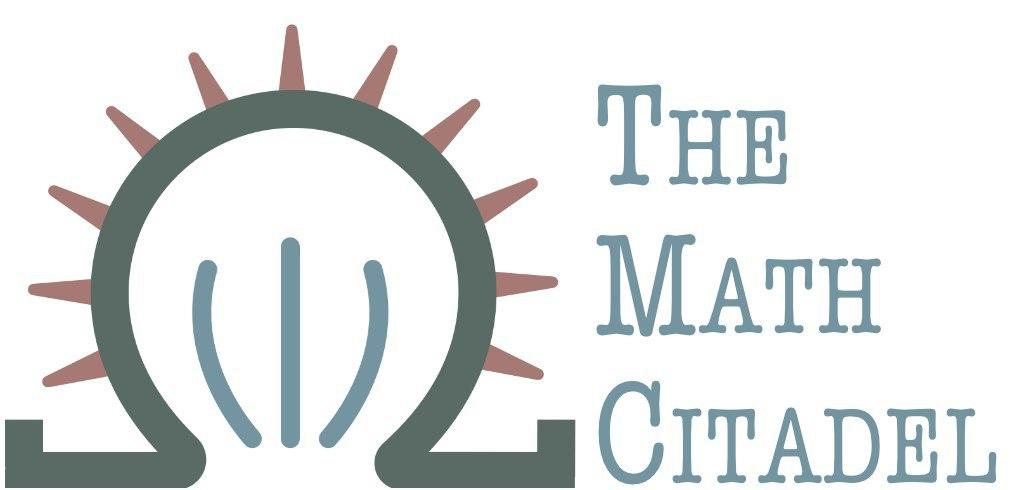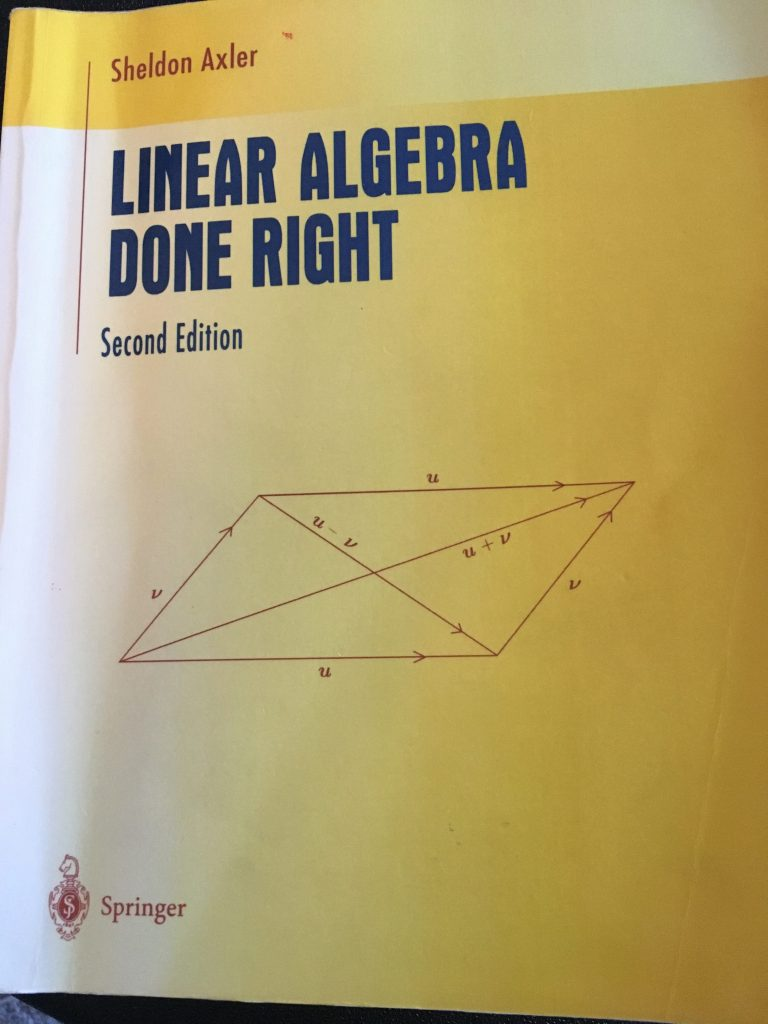## Book Review: Linear Algebra Done Right

### Author: S. Axler

#### Reviewed by: R. Traylor## Ranking

All ranking is done on a scale of 1-5, with 5 as the best.
Attribute Rank
Difficulty 2-5 (depending on chapter)
Proof Quality 3
Self-Study 2
Good for Teaching? 3
Quality of Exercises 3

## Topics Covered

• vector spaces introduction
• direct sums
• spanning sets, linear independence, basis, and dimensions
• linear maps
• null spaces, ranges
• matrix of a linear map
• invertibility
• eigenvalues and eigenvectors
• introduced via invariant subspaces
• polynomials on operators
• upper triangular matrices and invariant subspaces
• inner product spaces, norms, and orthonormal bases
• orthogonal projections
• linear functionals and adjoints
• self-anoint and normal operators
• the spectral theorem
• isometries
• singular-value decompositions
• generalized eigenvectors, nilpotent operators, and the characteristic polynomial
• minimal polynomial
• Jordan basis
• trace and determinant of a matrix